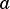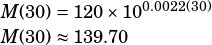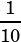Can you explain this algebraically (even if you also give a graphed explanation)?

Radioactive substances decay over time. the mass M, in grams, of a particular radioactive substance d days after the beginning of an experiment is shown in the table below:

Number of days, d | Mass, M (grams)
0 | 120.00
30 | 103.21
60 | 88.78
90 | 76.36

If this relationship is modeled by the function M(d) = a • 10^bd, which of the following could be the values of a and b?

A) a = 12 and b = 0.0145

B) a = 12 and b = -0.0145

C) a = 120 and b = 0.0022

D) a = 120 and b = -0.0022

The formatting of this question didn’t come through very well, but I think the function is, right?

First, plug in 0 forto see thatmust be 120. Remember, anything to the zero power equals 1!From there, you could ponder whether you want a positive or negative fractional exponent, or you could just try both and see which one gives you a value you expect from the table!Well, there you have it, D is the answer.

Now, why? Well, because when you have a negative exponent on 10, that’s the same as having a positive exponent on. As the exponent increases (which it will as days increase) the fraction gets smaller and smaller. That’s the behavior you want here as mass decays.Robin says: